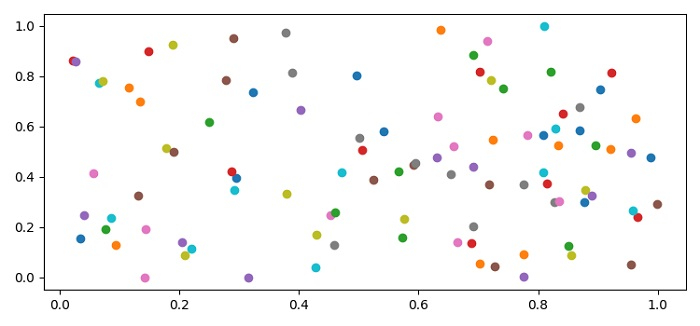# Python Scatter Plot with Multiple Y values for each X

MatplotlibPythonData Visualization

#### Python Data Science basics with Numpy, Pandas and Matplotlib

Most Popular

63 Lectures 6 hours

#### Data Visualization using MatPlotLib & Seaborn

11 Lectures 4 hours

#### MatPlotLib with Python

9 Lectures 2.5 hours

To make a scatter plot with multiple Y values for each X, we can create x and y data points using numpy, zip and iterate them together to create the scatter plot.

## Steps

• Set the figure size and adjust the padding between and around the subplots.

• Create random xs and ys data points using numpy.

• Zip xs and ys. Iterate them together.

• Make a scatter plot with each x and y values.

• To display the figure, use show() method.

## Example

import numpy as np
from matplotlib import pyplot as plt
plt.rcParams["figure.figsize"] = [7.50, 3.50]
plt.rcParams["figure.autolayout"] = True
xs = np.random.rand(100)
ys = np.random.rand(100)
for x, y in zip(xs, ys):
plt.scatter(x, y, cmap="copper")
plt.show()

## Output